# Solution assignment 05 Quadratic functions and graphs

### Assignment 5

The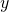-coordinate of the top of the parabola: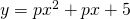is equal to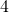.

Calculate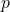.

### Solution

The-coordinate of the top can be calculated from: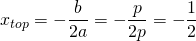We find the corresponding-coordinate by substituting this-coordinate in the equation of the parabola: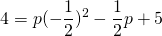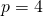0